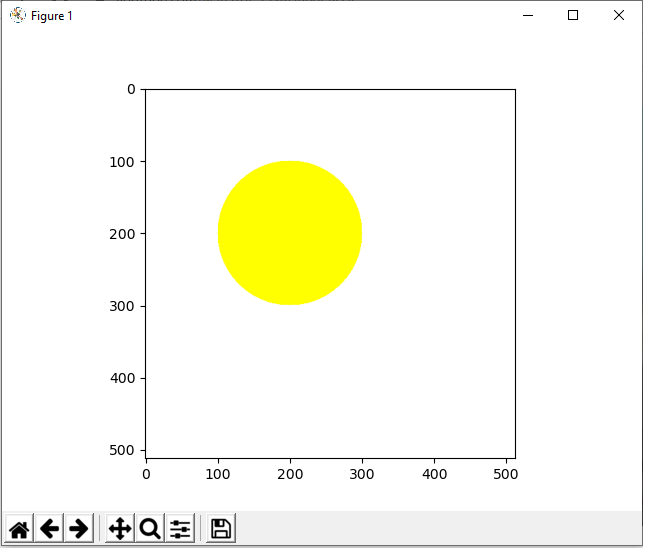﻿ How to Draw a Circle in Python using OpenCV ﻿# How to Draw a Circle in Python using OpenCVIn this article, we show how to draw a circle in Python using the OpenCV module.

OpenCV allows a user to create a wide variety of shapes, including rectangles, squares, circles, etc.

Python has a built-in circle() function, which allows us to add a circle to an image, usually a blank one. We create this blank image with numpy. Then using OpenCV, we add our circle shape to it.

There are many parameters that go into the circle() function, which allow us to control various aspects of it.

This includes its location (where it is on the blank image such as to the left, to the right, middle, upper, lower, etc.). It also includes its size (radius attribute), color, border thickness, and whether it's filled or unfilled.

In this article, we're going to create 2 types of circles, one where the center is unfilled and one where the center is filled.

### How to Create an Unfilled Circle

So the first type of circle we will create is one where the center is unfilled.

The circle we will create is shown below.The code to create this circle which is shown above is shown below.

Let's now go over this code.

First, we import all the modules we need which is cv2 (OpenCV), numpy (to create a blank image), and matplotlib (to get grided axes).

Next, we create a blank white image and store this in the variable, whiteblankimage. This is done using numpy.

We now have a blank image (like a canvass) in which we can now draw our circle.

Next, we use the cv2.circle() function to create our circle on this white blank image.

The first parameter that we must feed into the cv2.circle() function is the image we want to draw it on. We will be using what we created with numpy, whiteblankimage. This provides a perfect clean white background, in which we can place our circle shape.

The second parameter we must specify is where the circle is. We specify where the center of the circle is with the center attribute. We want our circle, in this example, to begin at the point, (200,100). If you're not sure where to begin, show the blank image with grids first before beginning the circle. Then you can see the dimensions of where you want this circle to be placed.

The third parameter we must specify is the length of the circle, which is done through the radius attribute. The radius is half the distance from the center to one end of the circle. In this example, we set our radius to 100. Since our center is located at (200,100), this means the left end of the circle will be (100,100) and the right end will be (300,100). The diameter of the circle then is 200.

Next, in the fourth parameter, we must specify the color of the circle. We specify the color with the RGB format. Since in this example our circle is yellow, this is represented by (255,255,0).

In the last parameter, the fifth parameter, we specify the thickness of the border of the circle. In this case, it's 10, which is relatively thick for a border.

### How to Create a Filled Circle

Next, we show how to create a circle whose interior is filled.

With no dimensions changed from the first image, the only change being that the interior is filled with color, this produces the following image shown below.The only difference between this circle and the other unfilled circle shown earlier is this circle is the thickness parameter of the cv2.circle() function.

To make a filled circle or any shape in Python, set the thickness attribute to -1.

The full code to this is shown below.

And this is how to draw a circle, unfilled or filled, in Python using the OpenCV module.

Related Resources